Free Printable Worksheets Covering Addition and Subtraction with Whole Numbers and Fractions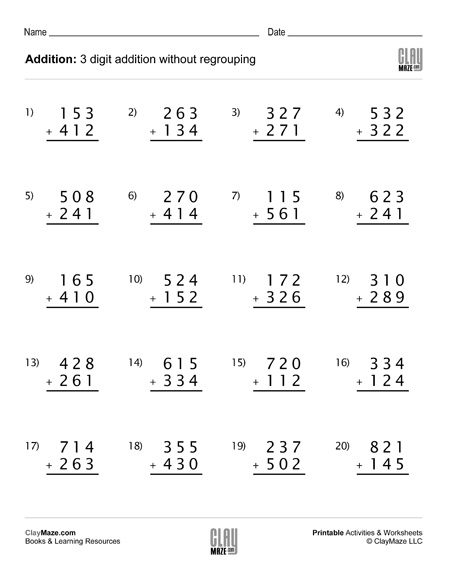## Subtraction Worksheet – 3 Digit Subtraction Without Regrouping (Set A)Download our free printable 3 digit subtraction worksheet with no regrouping.  20 subtraction problems – recommended for 1st grade...

## Subtraction Worksheet – 3 Digit Subtraction Without Regrouping (Set C)This worksheet has 20 subtraction problems with 3 digit numbers. There is no regrouping.  – Recommended for first grade and second grade....

## Subtraction Worksheet – 3 Digit Subtraction Without Regrouping (Set D)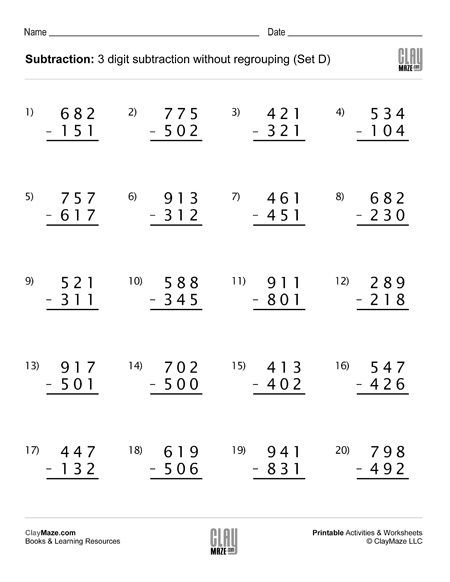Print this worksheet for subtraction practice with 3 digit numbers, without regrouping. Recommended for first grade and second grade....This one is a blank addition chart. Not only does this provide practice in addition, but also in using a table and understanding rows and columns....

## Subtraction Worksheet – 3 Digit Subtraction Without Regrouping (Set B)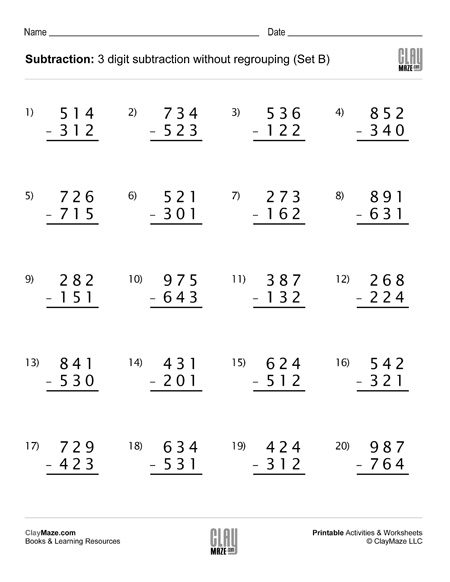3 digit subtraction worksheet – no regrouping, no borrowing. Set of 20 subtraction problems for lots of math practice....

## Fraction Addition with Same Denominators (Set 1)Math worksheet – fractions with the same denominators. Write the fractions below the pictures and add them. Color the sum in the picture and write the correct fraction under it....

## Fraction Addition with Same Denominators (Set 2)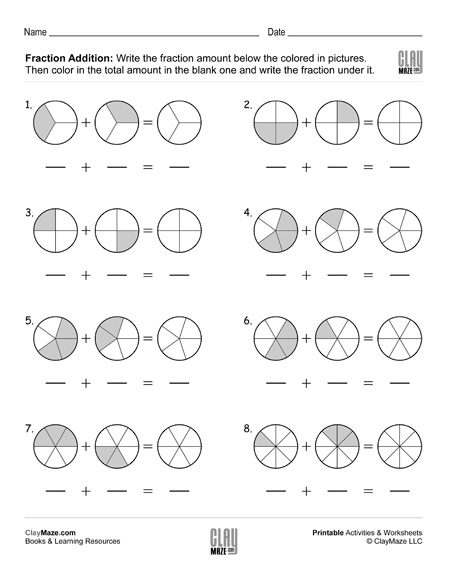Fractions worksheet with same denominators. Write the fraction amounts under the pictures. Color the total fraction amount in the blank picture and write the sum under it....

## Fraction Addition with Same Denominators (Set 3)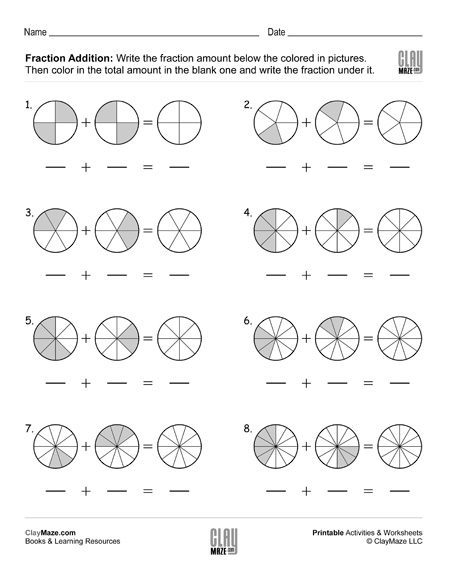Adding fractions with common denominator worksheet. Add the fractions and color the sum in the picture....

## Fraction Addition with Same Denominators (Set 4)Fraction worksheet – Write the fraction amount under he picture. Then color in the total fraction amount and write the sum in the blank below it....

## Fraction Addition with Same Denominators (Set 5)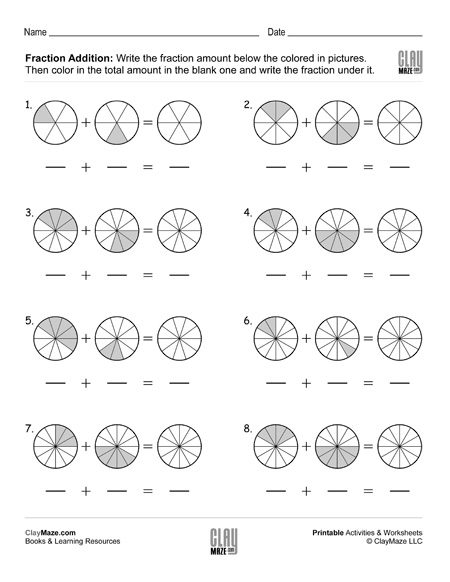Fraction math: addition – Write the fraction amounts below the pictures. Color in the total amount in the blank and write the amount below it.  ...

## Fraction Subtraction with Same Denominators (Set 1)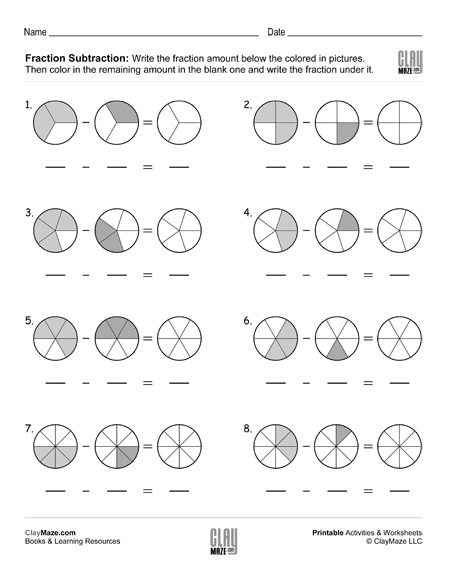Subtracting fractions worksheet – Write the fraction amount below the picture. Subtract the fractions and color in the remaining amount in the picture....